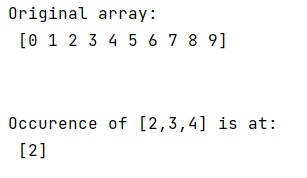# How to find the first occurrence of subarray in NumPy array?

Given a NumPy array, we have to find the first occurrence of a given subarray in it.
Submitted by Pranit Sharma, on March 12, 2023

## Finding the first occurrence of subarray in NumPy array

A subarray is part of an array that contains a random set of elements in the original sequence. For example, for an array [1,2,3,4,5], the sub-arrays can be [1,2,3], [2,3,4], etc. Note that [1,3,5] cannot be a subarray for this array.

We need to find the first occurrence of a subarray (say [2,3,4]), note that we need to get the index where the subarray starts. There may be similar subarrays at other positions but we want the first occurrence only.

To find the first occurrence of the subarray, there is a straightforward approach is to use the rolling window technique to search for windows of the appropriate size. This approach is simple, works correctly, and is much faster than any pure Python solution. It should be sufficient for many use cases. We will define a function that will accept the input array and the size of the subarray we want to search and it will return an array of boolean values with only True values at that position where the subarray occurs. We need to turn this array into a single index by using numpy.all() and numpy.mgrid() function.

Let us understand with the help of an example,

## Python code to find the first occurrence of subarray in NumPy array

```# Import numpy
import numpy as np

# Creating a numpy array
arr = np.arange(10)

# Display Original array
print("Original array:\n",arr,"\n\n")

# Defining a function
def fun(arr,size):
shape = arr.shape[:-1] + (arr.shape[-1] - size + 1, size)
strides = arr.strides + (arr. strides[-1],)
return np.lib.stride_tricks.as_strided(arr, shape=shape, strides=strides)

# Calling the function and Reducing the array
# along axis 1 and getting the exact index
inds = np.all(fun(arr, 3) == [2, 3, 4], axis=1)
res = np.mgrid[0:len(inds)][inds]

# Display result
print("Occurence of [2,3,4] is at:\n",res)
```

### OutputLanguages: » C » C++ » C++ STL » Java » Data Structure » C#.Net » Android » Kotlin » SQL
Web Technologies: » PHP » Python » JavaScript » CSS » Ajax » Node.js » Web programming/HTML
Solved programs: » C » C++ » DS » Java » C#
Aptitude que. & ans.: » C » C++ » Java » DBMS
Interview que. & ans.: » C » Embedded C » Java » SEO » HR
CS Subjects: » CS Basics » O.S. » Networks » DBMS » Embedded Systems » Cloud Computing
» Machine learning » CS Organizations » Linux » DOS
More: » Articles » Puzzles » News/Updates